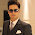# Define Equivalence Partitioning with Examples

## What is Equivalence Partitioning ?

The technique is to divide (i.e. to partition) a set of test conditions into groups or sets that can be considered the same (i.e. the system should handle them equivalently), hence ‘equivalence partitioning’. Equivalence partitions are also known as equivalence classes – the two terms mean exactly the same thing.

### Example 1 for Equivalence partitioning :

Test cases for input box accepting numbers between 1 and 1000 using Equivalence Partitioning:
1) One input data class with all valid inputs. Pick a single value from range 1 to 1000 as a valid test case. If you select other values between 1 and 1000 then result is going to be same. So one test case for valid input data should be sufficient.

2) Input data class with all values below lower limit. I.e. any value below 1, as a invalid input data test case.

3) Input data with any value greater than 1000 to represent third invalid input class.

### Example 2 for Equivalence partitioning :

For example in a savings Bank account,
3% rate of interest is given if the balance in the account is in the range of \$0 to \$100,
5% rate of interest is given if the balance in the account is in the range of \$100 to \$1000,
And 7% rate of interest is given if the balance in the account is \$1000 and above.

We would initially identify three valid equivalence partitions and one invalid partition as shown below. [Click on the image for Zoom view]

### Example 3 for Equivalence partitioning :

A store in city offers different discounts depending on the purchases made by the individual. In order to test the software that calculates the discounts, we can identify the ranges of purchase values that earn the different discounts. For example, if a purchase is in the range of \$1 up to \$50 has no discounts, a purchase over \$50 and up to \$200 has a 5% discount, and purchases of \$201 and up to \$500 have a 10% discounts, and purchases of \$501 and above have a 15% discounts.

Now we can identify 4 valid equivalence partitions and 1 invalid partition as shown below:

 Invalid Partition Valid Partition(No Discounts) Valid Partition(5%) Valid Partition(10%) Valid Partition(15%) \$0.01 \$1-\$50 \$51-\$200 \$201-\$500 \$501-Above

Happy Sharing! :)

#### 2 comments :

1.It is really a great work and the way in which u r sharing the knowledge is excellent.
Thanks for helping me to understand basic concepts. As a beginner in software testing your post help me a lot.Thanks for your informative article. software testing Training

2.Nice Post. Thanks for the article.
Advanced Excel Coaching Classes in Bangalore

Share your feedback and queries here. Your feedback are more valuable to us!